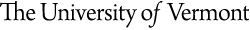#University of Vermont

|## Theoretical Condensed Matter Physics

Physics 128 Course Outline
Physics 128: Waves and Quanta
Course Outline

• Introduction to special relativity
1. Lorentz transformations
2. Length contraction, time dilation
3. Momentum-energy
4. Intro to general relativity
• Waves as particles
1. Photoelectric effect
2. Compton effect
• Particles as waves
1. Double-slit interference
2. Schrodinger equation
3. Uncertainty principle
4. Bohr model
• Bound states
1. Particle in a box
2. Finite well
3. Harmonic oscillator
• Unbound states
1. Potential step
2. Potential barrier and tunneling
• Introduction to quantum mechanics
1. Schrodinger equation in 3D
2. 3D infinite well
3. Hydrogen atom
• Atomic physics
1. Identical particles
2. Exclusion principle
3. The periodic table
4. Spin-orbit interaction
• Statistical mechanics
1. Entropy and temperature
2. Boltzmann statistics
3. Quantum statistics
4. Photon gas
• Molecules and solids
1. Crystalline solids
2. Energy bands
3. Semiconductors
4. Superconductors